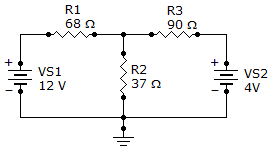# What is the current through R2?A. 3.19 A

B. 319 mA

C. 1.73 A

D. 173 mA

Related Questions on Branch, Loop and Node Analyses

The branch current method uses.

A. Kirchhoff's voltage and current laws

B. Thevenin's theorem and Ohm's law

C. Kirchhoff's current law and Ohm's law

D. The superposition theorem and Thevenin's theorem